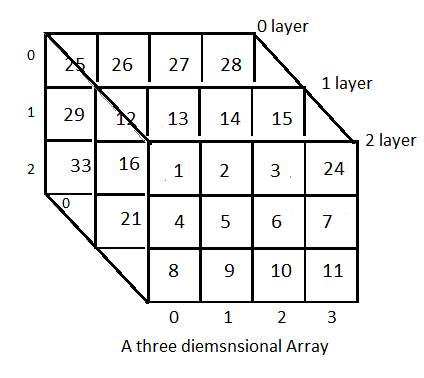# Types of Arrays

Types of arrays depend upon the number of dimensions of an array. The count of indices or subscripts required to access one element of an array define the dimensions of an array .

## Types of arrays

• One-dimensional Array
• Two-dimensional Array
• Three-dimensional Array

Two dimensional and three dimensional arrays are also called multi-dimensional arrays. in types of arrays, multi-dimensional arrays also include arrays with four and higher dimensions.

## One-dimensional Array

In a one-dimensional array the elements are stored in contiguous memory locations where each element is accessed by using a single index value. It is a linear data structure storing all the elements in sequence.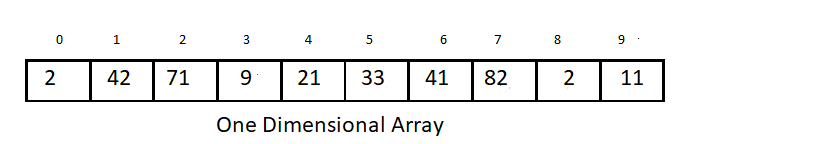The elements are stored in memory in continuation and the variable declared as an array is actually a pointer to the address of first element of the array. This address is called the base address. The address of any other element can be calculated with the following formula

Where

• ADDRESS -memory location of the Kth element of the array (To be calculated)
• ARRAY – name of the ARRAY
• WORDLENGTH – number of bytes required to store one element depending upon its data type like a character value needs 1 byte and an integer value needs 2 bytes.
• LOWERBOUND – index of the first element of the array.
•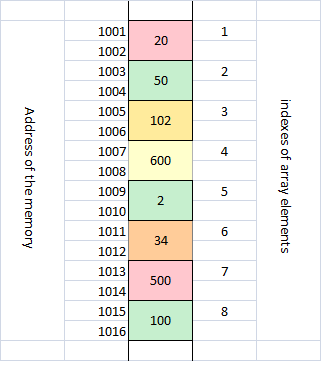In this example a snap shot of the memory displays how an array of size 8 is stored in memory. To calculate memory address of a given element say 6th element of the array you will put the values in the previous formula

K=6

WORDLENGTH=2 (integer data)

LOWERBOUND =1 (index of first element of the array)

Putting these values in formula

ADDRESS(ARRAY)= 1001+ 2*( 6-1)  =1011 this is the address of memory location where 6th element (34) is stored as visible in the figure above

## Two-dimensional Array

In types of arrays, a two dimensional array is a tabular representation of data where elements are stored in rows and columns. A two dimensional array is actually a collection of M X N elements which has M rows and N columns.  To access any element in a two-dimensional array two subscripts are required for defining position of an element in a specific row and column. The first index is for row number and second is for column index.  In the example shown the first index values row=2 column=3 is used to access element 56.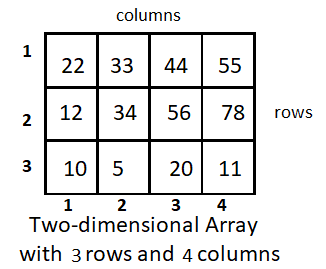Two dimensional arrays are stored in memory in two representations

### Row Major Representation

In the row major representation the storage of array elements takes place row wise. All elements of first row of the array are first stored in sequence followed by second row and then third, fourth and so on. The 2-dimensional array given in previous examples is stored in row-major representation in the figure below.

To find the Address of any element located at Ith row and Jth column is calculated by using the formula

Where

• ADDRESS – memory location of the element at  Ith row and  Jth  columnof the array (To be calculated)
• ARRAY – name of the ARRAY
• WORDLENGTH – number of bytes required to store one element depending upon its data type like a character values needs 1 byte and an integer value needs 2 bytes.
• N – number of columns of the array.

In this example to calculate memory address of a given element (44) says in 1st row and 3rd column you will put the values in the formula.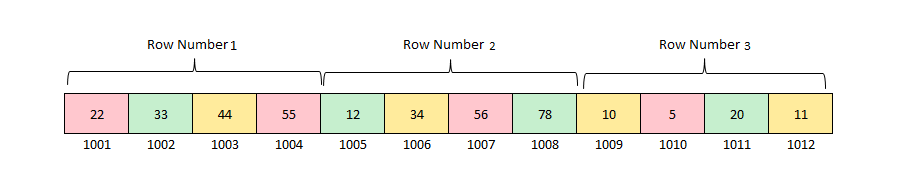I=1, J=3

N=4

WORDLENGTH=1 (assuming only one byte is required to store these small ints )

ADDRESS(ARRAY(6))= 1001+ 1*( 4*(1-1) + (3-1)) =1003 this is the address of memory location where 44 is stored as visible in the previous figure

### Column Major Representation

In the column major representation the storage of array elements takes place column wise. All elements of first column of the array are first stored in sequence followed by second column and then third, fourth and so on. The 2-dimensional array given in previous examples is stored in column-major representation in the figure below.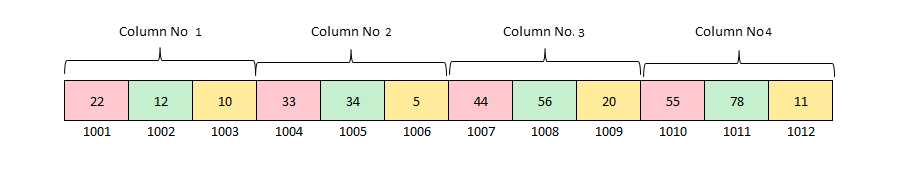To find the Address of any element located at Ith row and jth column is calculated by using the formula

• ADDRESS – memory location of the Ith and  Jth element of the array (To be calculated)
• ARRAY – name of the ARRAY
• WORDLENGTH – number of bytes required to store one element depending upon its data type like a character values needs 1 byte and an integer value needs 2 bytes.
• M – number of rows of the array.

In this example to calculate memory address of a given element (44) say in 1st row and 3rd column you will put the values in the formula.

I=1, J=3

M=3

WORDLENGTH=1 (assuming only one byte is required to store these small ints )